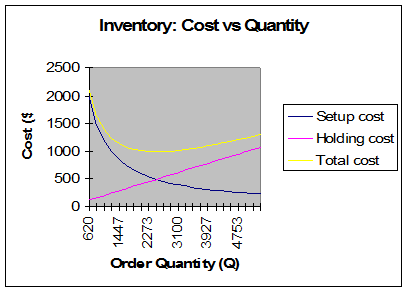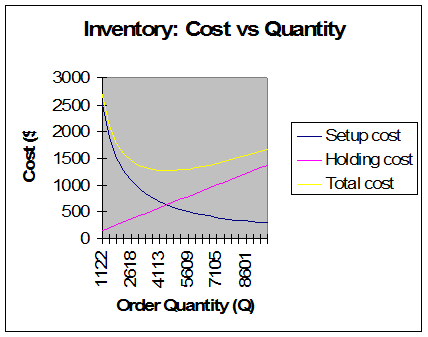# Learn How to Find Optimal Inventory Levels. Operations Management Help

 We offer quality and prompt Operations Management Homework Help We can help you with any kind of Operations Management assignments, homeworks, tests, exams, at any level Including topics such as Forecasting, PERT, Aggregate Planning, Waiting Lines, Network Problems, Linear Programming and Transportation Problems, Inventory, Quality Control and much more.

${{Q}^{*}}=\sqrt{\frac{2DS}{H}}=\sqrt{\frac{2\times 15,000\times 82}{0.40}}=2,479.919\approx 2,480$
 Data Demand rate, D 15000 Setup cost, S 82 Holding cost, H 0.4 Unit Price, P 40 Daily demand rate 41.09589041 Lead time in days Results Optimal Order Quantity, Q* 2479.919354 Maximum Inventory 2479.919354 Average Inventory 1239.959677 Number of Setups 6.048583789 Holding cost $495.98 Setup cost$495.98 Unit costs $600,000.00 Total cost, Tc$600,991.97 Reorder Point 0$TC=\frac{D}{{{Q}^{*}}}S+\frac{{{Q}^{*}}}{2}H=495.98$ $TC=\frac{D}{Q}S+\frac{Q}{2}H=1430$
 Inventory Production Order Quantity Model Data Demand rate, D 5700 Setup cost, S 500 Holding cost, H 0.4 Daily production rate, p 78 Daily demand rate, d 22.8 Unit price, P 48 Results Optimal production quantity, Q* 4487.301 Maximum Inventory 3175.628 Average Inventory 1587.814 Number of Setups 1.270251 Holding cost 635.1257 Setup cost 635.1257 Unit costs 273600 Total cost, Tc 274870.3

${{Q}^{*}}=\sqrt{\frac{2DS}{H\left( 1-\frac{D}{P} \right)}}=\sqrt{\frac{2\times 5,700\times 500}{0.4\left( 1-\frac{5700}{195\times 100} \right)}}=4,487.301$.